### Home | Up One Level | Previous | Next

WALTER RITZ

#### Annales 252 (Oeuvres 406)

While concerning, as in the preceding sections, the hypothesis that the speeds are small in relation to c, we are going to assume the acceleration to be very great; such phenomenon will be either of very short duration or oscillatory. In hertzian oscillations, the speeds, corresponding to weak currents, will scarcely exceed values on the order of 1 cm per second and the distance where one may study them may be evaluated to a very small number

of wavelengths. The number of oscillations per second varies from 108 (corresponding to 3 meters of wavelength) to 1011. As to the amplitudes of the electron [oscillations], they are extremely small, as the speeds remain small despite the very great frequencies. Under these conditions, the quantityis always very small, and the case which has been in question doesn’t present itself. Indeed, let us suppose the vibrations to be sinusoidal, and let | v'| be the maximum value of v', | w'r| that of w'r, n the number of oscillations per second, (Oeuvres 407) mλ the maximum distance where we may observe the waves, m is scarcely on the order of 10 to 100. We will have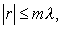be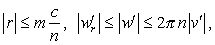from where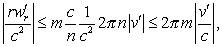a number, we have seen, comparable to 10-9 or 10-8.

We have designated by R the vector whose components are x-x', y-y', z-z'. We have thus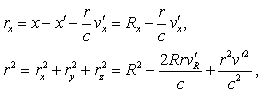from which follows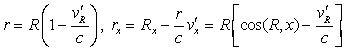to the terms in about. The first term of (31) is therefore written.

This expression is very clearly identical to the first term of (33). Indeed, in Lorentz’s theory, the center of disturbance remains fixed in relation to the exciter and to the resonator, when, as we suppose, the latter are immobile in relation to the ether (the material movements are besides infinitely slow in relation to the phenomena in question). This center disturbance therefore coincides with the position of x', y', z' at a certain moment; but the amplitude of the movements of the electrons being extremely small, the quantities Rx, Ry, Rz, R are very clearly constant. The electrostatic terms are therefore obviously identical in both theories. Besides, the ratios of sizes and direction of r and of R differ only (Oeuvres 408) in quantities of the order of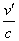, that is 10-10; one may thus certainly replace r and R by each other in the second terms of formulas (31) and (33), and even in the argument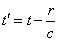; this last hypothesis comes back to identify the speeds of propagation and the phases which are between them in the ratio of 1 to 1+10-10, that is differing only in a quantity which these experiments can not show.

In summary, both theories lead, for hertzian oscillations, to exactly the same results.